Study Guide

# Graphs of Linear Equations

## Graphs of Linear Equations

Tired of plugging and chugging random numbers whenever you want to graph the equation of a line? Wish there were a way to easily find the equation of a line from its graph? If you're sick of all the hassle, then do we have a solution for you.

Whoa, we totally sounded like a grade-A TV infomercial there for a second. But it's true; we have not one, but two ways to work with lines and their graphs. Oops, we did it again.

• ### Slope-Intercept Form

Now that we’ve totally sold you on these two hassle-free ways of graphing lines, let us teach you how each method works. We promise, these are not rip-offs like some infomercials. Why did we ever buy that potato peeler that doubles as a laser pointer?

The first method is the slope-intercept form. It looks like this:

y = mx + b

We know that m is the slope of the line, but what is b? We may not be a world-class detective, but we're thinking it's an intercept of some kind. It turns out that b is the y-intercept specifically: (0, b).

If we're given an equation in slope-intercept form, we immediately have the slope and one point on the line, making it a cinch to graph. Alternatively, if we know m and b, we can quickly create an equation for the line. This form is handy no matter which end we're starting from.

### Sample Problem

Graph the equation y = 2x + 1.

This is in slope-intercept form, so we know that the number in front of x is the slope, and that 1 is the y-intercept. Our brains are just bulging with knowledge, and we haven't even started yet.

Plot the y-intercept to start, at (0, 1):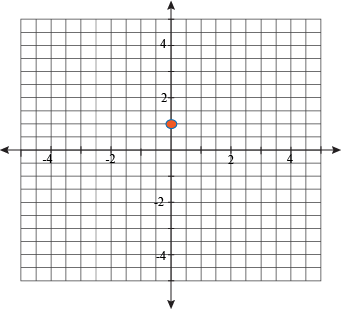Next, because we know that the slope is 2, also known as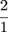, we know that another point will be two units up and one to the right. That puts us at (1, 3). Remember, the 2 may come first in the slope, but the rise is in the y-value, which is second in our point.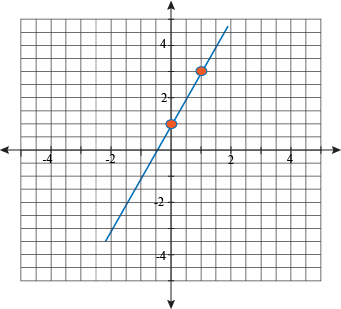How about we do one more, just to be safe?

### Sample Problem

Find the equation of the line for the following function.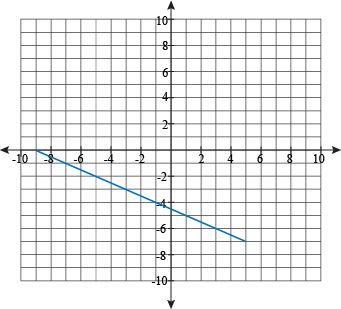Wait a second, "just to be safe"? That makes it sound like we would have been unsafe if we didn't do this problem. We didn't mean that as a threat, honest.

The y-intercept of this line is hanging out right in the middle of -4 and -5. So, b = -4.5. Now for the slope. Let's start at (-3, -3) and work our way over to (-1, -4). Both of them are on the line, and they have the distinction of not being fractions. Sorry, fractions, but you're hard to work with.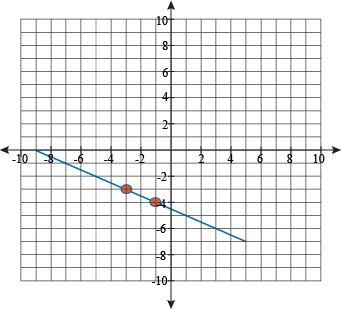Our rise is -1 and our run is 2. This gives us a slope of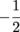. The pieces have all been gathered. Let's assemble them, Voltron style, into a space robot-fighting equation for the line.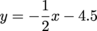Yes, this looks like an equation capable of taking on Zarkon. Totally.

• ### Standard Form

Don't walk away just yet; we still need to introduce you to the second half of the deal: equations of lines in standard form. Remember? We promised a two-for-the-price-of-one special.

### Sample Problem

Graph the line 2x – 3y = 4.

This equation is already in the standard form of a line. Standard form looks like this:

Ax + By = C

A, B, and C are all real numbers of some kind, but A and B are not both 0; if they were, we wouldn’t have an equation and that would be pointless.

While slope-intercept form lets us find the slope and y-intercept quickly, standard form gives us easy access to the x- and y-intercepts. Just plug in 0 for the other variable and solve. Like so:

2x – 3(0) = 4

2x = 4

x = 2

That's the x-intercept, (2, 0). Now plug in x = 0.

2(0) – 3y = 4

-3y = 4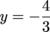And here's the y-intercept,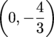. Now we have two easy-to-plot points at our disposal. No messing around with the slope, no rising and running, just two dots and a line and we're done.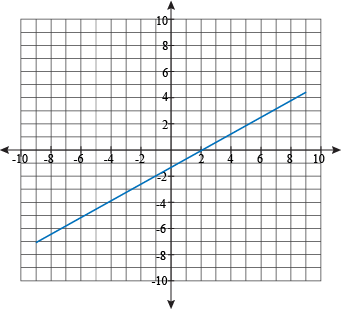### Sample Problem

Find the slope of the following line, then graph it.

-2xy = 6

Hey now, didn't we just say that we didn't have to mess with the slope anymore? What gives?

Put down the pitchforks and stop asking for a refund. Sometimes there are good reasons to find the slope of a line in standard form. For instance, what if we want to see if it's parallel or perpendicular to another line? That's a good reason, see?

There are three ways to go about finding the slope of a line in standard form. The first is to find the x- and y-intercepts, and then calculate the slope from them.

-2xy = 6

-2x – 0 = 6

x = -3

We have (-3, 0) for the x-intercept.

-2(0) – y = 6

y = -6

And (0, -6) is the y-intercept. Now we use the formula to find the slope. This is a lot faster than checking under every rock and inside every cabinet drawer.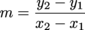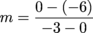At least there are plenty of zeros to make the calculations simple.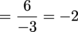Our first method found a slope of -2. The other two better find the same thing, or we'll be all kinds of grumpy.

The second method is to convert the equation to slope-intercept form. That may seem like a cop-out, but the slope-intercept form was practically custom-made for finding the slope.

-2xy = 6

-y = 2x + 6

y = -2x – 6

We again have a slope of -2. Good, good.

The last method for finding the slope is to graph the line and find it visually. We recommend skipping this method, unless we have some other reason to graph the line. Like we do now.

We already have the intercepts, (-3, 0) and (0, -6), and the slope, -2. We're way over-prepared for graphing this line.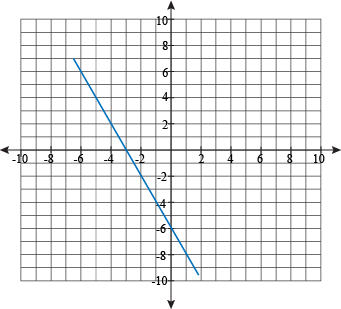Oh look, a slope of -2. Who would have guessed?